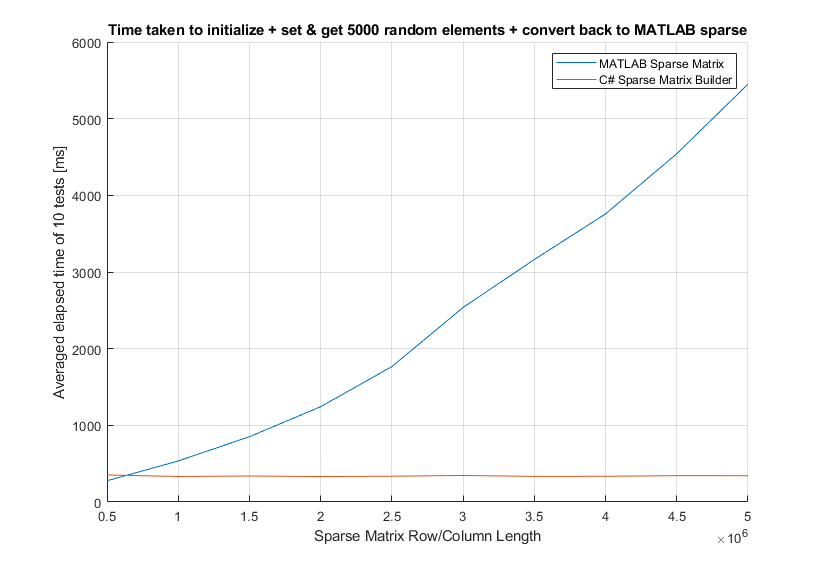## Fast Sparse Matrix Builder

version 1.0.4 (29.2 KB) by
Much faster routines for creating and build huge sparse matrices in MATLAB. Latest version on github (file exchange seems to not autoupdate)

Updated 23 Dec 2021

From GitHub

# Sparse-Matrix-Builder

### By C. Souto (csouto@fe.up.pt)

Much faster routines for creating and building huge sparse matrices in MATLAB.

Usage example:

```% reset workspace
clear all; close all; clc;

% load the .NET assembly
dll = [pwd '\SparseLibrary.dll'];

% create a sparse matrix builder
m = 8;
n = 4;
matrix = SparseMatrixBuilder(m, n);

% set some values
matrix.Set(1, 3, 5.0);
matrix.Set(2, 4, 6.0);
matrix.Set(7, 1, 4.0);
matrix.Set(7, 3, 9.0);
%matrix.Set(9, 3, 1.0); % Error: Index was outside the bounds of the array.

% set multiple values
matrix.Set([1 2 3], [4 3 2], [10. 20. 30.]);

% add some values (same as set, except the value is added to the matrix element, while set replaces it)
%matrix.Set(9, 3, 1.0); % Error: Index was outside the bounds of the array.

% add multiple values (same as set, except the value is added to the matrix element, while set replaces it)
matrix.Add([1 2 3], [4 3 2], [10. 20. 30.]);

% get some values (print)
matrix.Get(7, 1)
matrix.Get(1, 1)
%matrix.Get(8, 5) % Error: Index was outside the bounds of the array.

% get multiple values
matrix.Get([1 2 3], [4 3 2])

% set all values in the diagonal
matrix.SetDiagonal(5.0)

% get the diagonal (print)
matrix.GetDiagonal()

% set and get a row
matrix.SetRow(2, 6.0);
matrix.GetRow(2) % will print

% set and get a column
matrix.SetColumn(2, 8.0);
matrix.GetColumn(2) % will print

% to dense (print)
matrix.ToDense()

% to COO format (print)
matrix.ToCOO()

% to MATLAB sparse (print)
matrix.ToMatlabSparse()```### Cite As

Carlos Souto (2022). Fast Sparse Matrix Builder (https://github.com/carlosouto/Sparse-Matrix-Builder/releases/tag/v1.0.4), GitHub. Retrieved .

##### MATLAB Release Compatibility
Created with R2021a
Compatible with any release
##### Platform Compatibility
Windows macOS Linux

### Community Treasure Hunt

Find the treasures in MATLAB Central and discover how the community can help you!

Start Hunting!

#### MATLAB/Tests

To view or report issues in this GitHub add-on, visit the GitHub Repository.
To view or report issues in this GitHub add-on, visit the GitHub Repository.